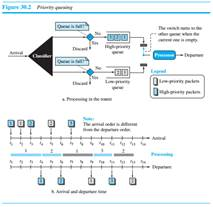Create an Account

Home / Questions / In Figure 30 3 assume the weight in each class is 4 2 and 1 The packets in the top queue a...

In Figure 30 3 assume the weight in each class is 4 2 and 1 The packets in the top queue are labeled A in the middle queue B and in the bottom queue C Show the list of packets transmitted in

In Figure 30.3, assume the weight in each class is 4, 2, and 1. The packets in the top queue are labeled A, in the middle queue B, and in the bottom queue C. Show the list of packets transmitted in each of the following situations:

a. Each queue has a large number of packets.

b. The numbers of packets in queues, from top to bottom, are 10, 4, and 0.

c. The numbers of packets in queues, from top to bottom, are 0, 5, and 10.Jul 29 2020 View more View LessSubscribe To Get Solution